top of page
Search

# Learn the Shape Maker. Lines shaping.

Since most of the lines in Shape Maker form a wire frame on which to build surface patches, the shape of the lines is important for building smooth surface patches. Editing a control polygon of B-spline curves is a procedure described many times in various instructions. Therefore, we will analyze only some of the features and techniques inherent in Shape Maker.Example of complicated curve.

## Repositioning the endpoints of lines.

By default, all input lines are straight. The easiest way to modify a straight line is to change the position of its end points. Since the Shape Maker is always in edit mode, just left-click on the edited point and move it to a new position and once again click to fix the point in a new position, as shown below.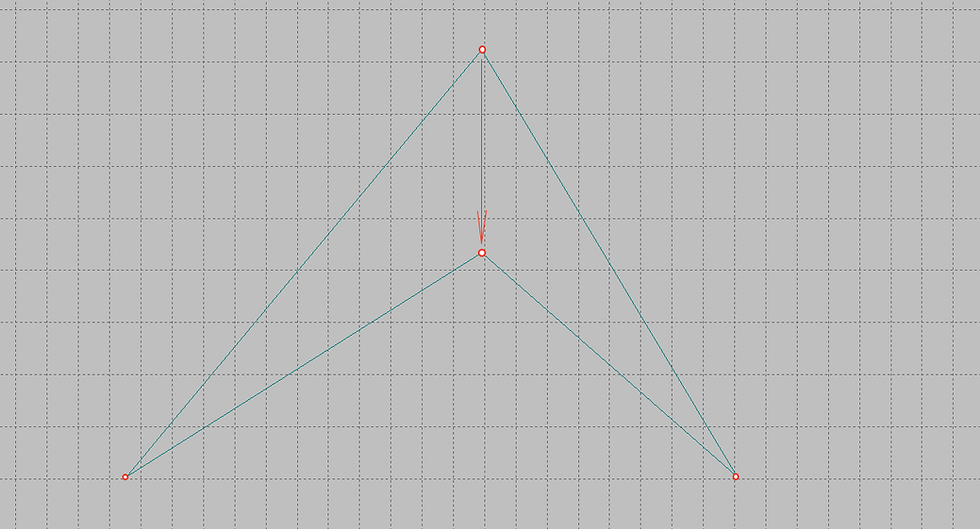Change endpoint position.

In this case, the editable point converges two topologically connected lines. Therefore, both lines followed the point when changing its coordinates.

## Editing a curve shape.

Very often, changing not only the position of its end points, but the shape of line itself is required. For this editing, just left-click on the line whose shape you want to change.Lines control polygon in edit mode.

The Shape Maker will show a control polygon for this line. Changing the shape of the control polygon will dynamically change the shape of the editable line. By default, a control polygon has two control points. The direction of the vector from the end point to the intermediate point of the polygon shows the angle of inclination of the curve to the end point, and the length of this vector indicates the degree of line fit to this vector at the end point. So if the vector is located horizontally or vertically, we will have a horizontal or vertical tangent at this point. Editing the points of the control polygon, you can try to achieve the desired shape of the curve.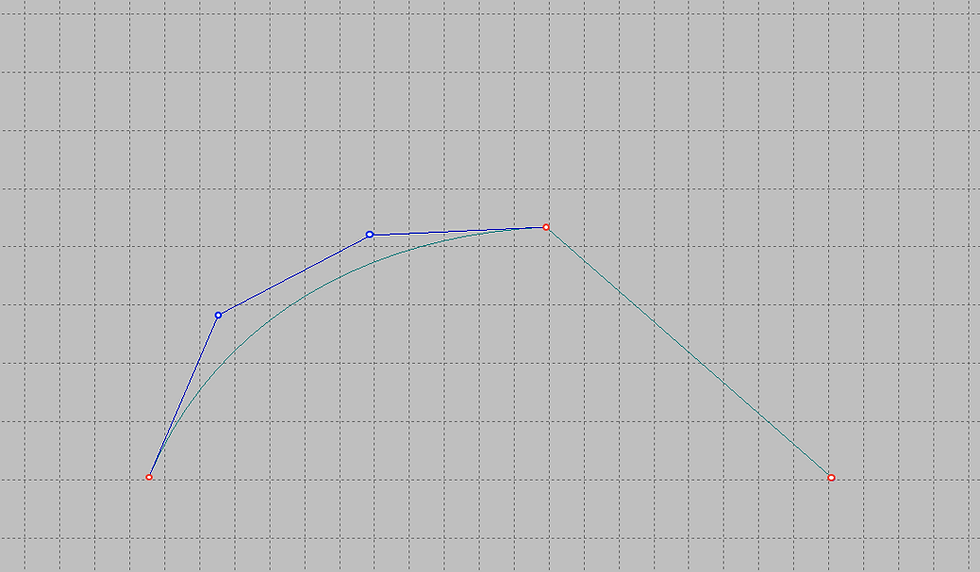Changing curve shape by moving points of control polygon.

## Change the number of points of a control polygon.

Often ship curves are more complex than the curve shown above. Describing such curves requires more degrees of freedom. In our case, more control points are required.

There are several ways to add additional points to the control polygon. The easiest way is to click the left mouse button while holding down Ctrl on the line connecting the control points to each other. The system will add an additional control point to the control polygon. In this case, the shape of the curve does not change. If you need to reduce the number of points you need to right-click, while simultaneously pressing Ctrl, on the line between the control points. This method allows you to add a certain number of control points from the following series: 4,5,7,11,19,35,67. The reasons for using this series will be explained later.

You can also add and remove an arbitrary number of control points using the following command.Or from the Modify toolbar:In the dialog box, using the marked options, specify the required number of control points.An additional control point will provide more opportunities for changing the shape of the curve.Now we can change the shape of the curve while maintaining the slope angles of the start and end points.Extra point in control polygon.Lines shape changes with keeping tangency in endpoints.

## Defining tangents at the end points of a curve.

Quite often, it becomes necessary to establish tangents at the start and end points of the line strictly horizontally or vertically.To do this, just left-click with the pressed Ctrl button on the line between the end point and the first control point of the polygon.In this case, the tangent will be set horizontally or vertically depending on the initial angle of inclination of the tangent at this point.If the angle of inclination is less than 45 °, the tangent will be horizontal, if more - vertical.Orthogonal tangents in endpoints.

The same can be done by calling the appropriate command from the menu.Or from the Modify toolbar:Note that in this case, the dialogue is organized somewhat specifically.Before invoking this command, it is necessary that the last point that the user edited is one of the points closest to the end point of the line.Otherwise, the following message appears.Simply put, the system needs to be made clear which of the tangents needs to be set horizontally or vertically.

## Smooth connection of curves.

Another problem often arises when it is necessary to perform smooth conjugation of a given curve with another having a common point. In this case, the user must first approximately set the angle of inclination of the tangent at this point, and then call the next command.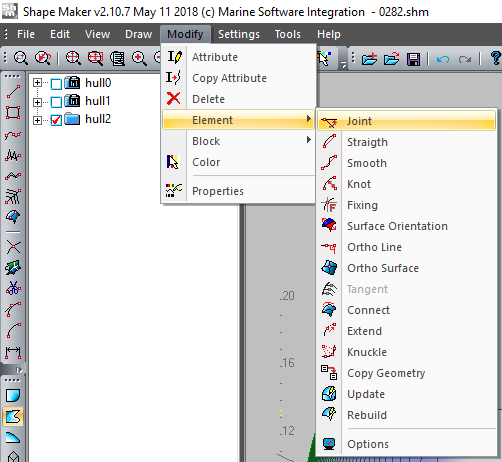Or from the Modify toolbar:Before usinf this command, it is necessary that the last point that the user edited is one of the points closest to the end point of the line.Otherwise, the following message appears.If the edited point is selected correctly, select the specified functions in the dialog box that appears and click OK.In this case, the tangent to the line will be set exactly.Smooth connection between two lines.

The Shape Maker allows joining curves both in space and on one projection.Docking with a surface and with a plane formed by two lines is also possible.

## Straightening lines.

One of the advantages of this type of curve is the ability to straighten the entire curve or part of the curve. To do this, set a number of control points on one straight line. The easiest way to do this is by left-clicking with the Ctrl button pressed on the start and end points of the control polygon area that needs to be rectified. In this case, all the intermediate points of the selected area will be projected onto the line formed by the start and end points. The type of projection will be determined by the angle of inclination of this line to the horizon. If the angle is less than 45 °, the points will be projected vertically, if more - horizontally.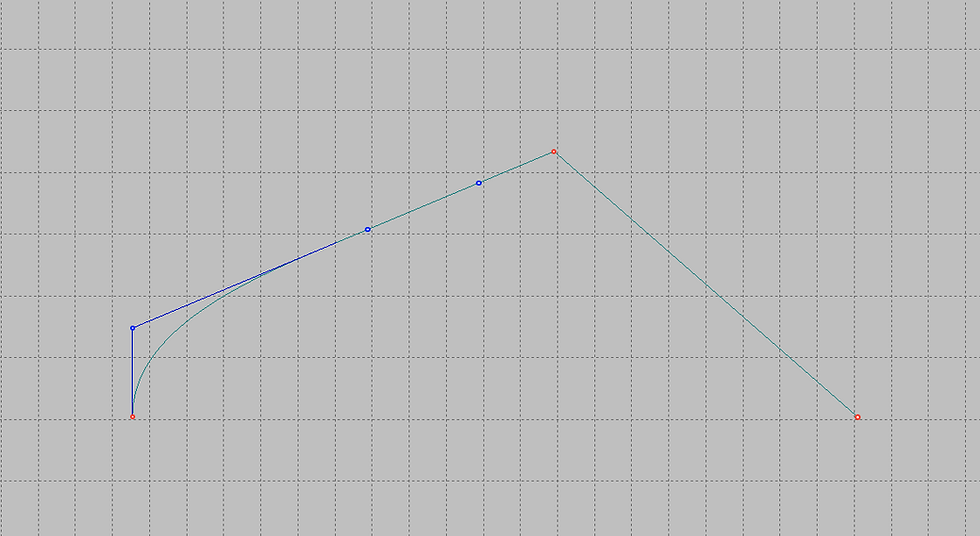Straightening part of line.

An extended variant of straightening curves can be used to call the following command from the menu.Or from the Modify toolbar:The curve straightening dialog describes almost all possible cases.## Editing a group of control points of a polygon.

Sometimes it becomes necessary to change several control points at the same time. The modification mode of the group of control points of the polygon is designed for this. By default, the mode for changing one control point is valid. In order to switch to the editing mode of a group of control points, just left-click the next window status bar.After that, set the number of points to change in the next bar status field.Moreover, if you left-click the number of points will increase, and if you right-click, decrease.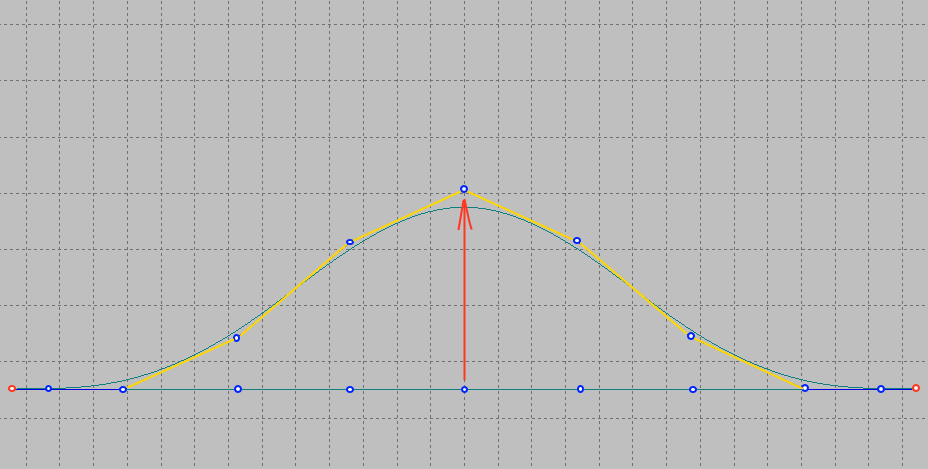Changes group of control points.

Modification of the group of control points depicted above was performed with the following values.As you can see in the image, the center point is moved by the cursor. Two points on the left and right follow the moved center point.For convenience, the control polygon, which changes when the center point is moved, is highlighted with yellow lines.

Enabling this mode can also be performed by the following command.Or from the Modify toolbar: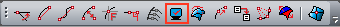And by selecting the marked mode in the dialog box that appears.## Modes of changing the shape of the curve when changing the position of its end point.

Since the objects in the system have topological connections and depend on each other, when the position of the control point changes, the curve must change its shape. There are three different modes in the system to change the shape of the curve when the position of its end point changes.You can set one of these modes with the command.Or from the Modify toolbar:And choose one of three possible options in the dialog box that appears.The same can be done by left-clicking in the next field of the status bar.One of the modification modes is always current. Let's consider how these modes affect the line shape.

- Mode without modifying the points of the control polygon of the curve.In this mode, when changing the position of the end point, all points of the control polygon of the curve remain in their places.Endpoint modification.

This mode is used when it is necessary to save the shape of the curve and only modify its end point.

- Modification mode of all control points of the curve.In this mode, changing the position of the control point will lead to a change in all points of the control polygon according to a linear law.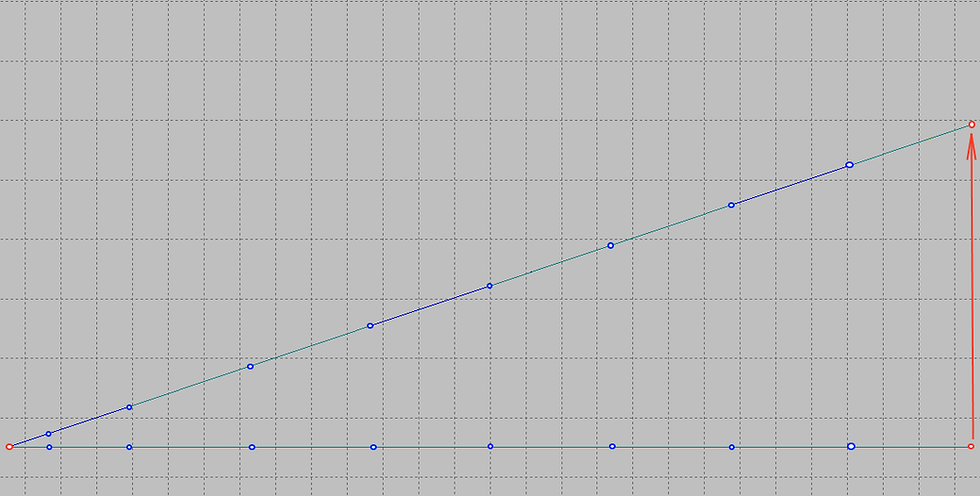Endpoint modification.

This mode is convenient to use when you want to change the entire curve without paying attention to the tangents at the end points.

- The modification mode of all control points of the curve except for the control points closest to the end points.In this mode, the tangents do not change at the start and end points. All other intermediate points are recalculated linearly.This mode is convenient to use if you want to save the value of the tangent curve of the end points. For example, in order to maintain smooth conjugation of two curves at an editable point.

The last two of the above modes can work together with the modification mode of part of the control points of the curve.In this case, only that part of the curve that is indicated in the modification mode of the curve region is modified.